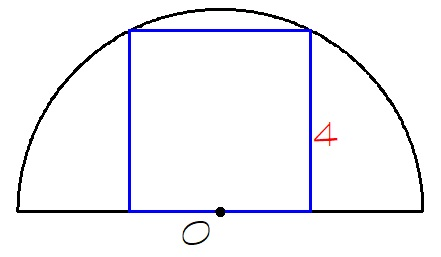Square in a semicircle

Geometry Level 2A square of perimeter $16$ is inscribed in a semicircle, as shown.

Find the perimeter of the semicircle rounded to the nearest integer.

Use $\frac{22}{7}$ for the approximation of $\pi$.

×